Tamil Nadu 12th Chemistry Model Question Paper 2 English Medium

Students can Download Tamil Nadu 12th Chemistry Model Question Paper 2 English Medium Pdf, Tamil Nadu 12th Chemistry Model Question Papers helps you to revise the complete Tamilnadu State Board New Syllabus and score more marks in your examinations.

TN State Board 12th Chemistry Model Question Paper 2 English Medium

Instructions:

1. The question paper comprises of four parts
2. You are to attempt all the parts. An internal choice of questions is provided wherever: applicable
3. All questions of Part I, II, III, and IV are to be attempted separately
4. Question numbers 1 to 15 in Part I are Multiple choice Questions of one mark each.  These are to be answered by choosing the most suitable answer from the given four alternatives and writing the option code and the corresponding answer
5. Question numbers 16 to 24 in Part II are two-mark questions. These are lo be answered in about one or two sentences.
6. Question numbers 25 to 33 in Part III are three-mark questions. These are lo be answered in about three to five short sentences.
7. Question numbers 34 to 38 in Part IV are five-mark questions. These are lo be answered in detail. Draw diagrams wherever necessary.

Time: 3 Hours
Maximum Marks: 70

Part-I

Answer all the questions. Choose the correct answer. [15 × 1 = 15]

Question 1.
The following set of reactions are used in refining Zirconium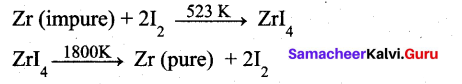This method is known as ……………..
(a) Liquation
(b) Van Arkel process
(c) Zone refining
(d) Mond’s process
(b) Van Arkel process

Question 2.
The stability of+1 oxidation state increases in the sequence ……………
(a) Al < Ga < In < Tl
(b) Tl < In < Ga < Al
(c) In < Tl < Ga < Al
(d) Ga < In < Al < Tl
(a) A1 < Ga < In < TlQuestion 3.
Assertion : bond dissociation energy of fluorine is greater than chlorine gas.
Reason: chlorine has more electronic repulsion than fluorine.
(a) Both assertion and reason are true and reason is the correct explanation of assertion
(b) Both assertion and reason are true but reason is not the correct explanation of assertion
(c) Assertion is true but reason is false
(d) Both assertion and reason are false
(d) Both assertion and reason are false

Question 4.
Which of the following pair has d10 electrons?
(a) Ti3+ , V4+
(b) CO3+ , Fe2+
(c) Cu+, Zn2+
(d) Mn2+, Fe3+
(c) Cu+, Zn2+

Question 5.
Which is used for the separation of lanthanides, in softening of hard water and also in removing lead poisoning?
(a) [Ni(CO)4]
(b) EDTA
(c) [Ni(DMG)2]
(d) TiCl4 + Al (C2H5 )3
(b) EDTA

Question 6.
The yellow colour in NaCl crystal is due to ……………..
(a) excitation of electrons in F centers
(b) reflection of light from CF ion on the surface
(c) refraction of light from Na+ ion
(d) all of the above
(a) excitation of electrons in F centersQuestion 7.
For a reaction Rate = k[acetone] then unit of rate constant and rate of reaction respectively is
(a) (mol L-1 s-1),(mol-1/2 L1/2 s-1)
(b) (mol-1/2 L-1/2 s-1) , (mol L-1 s-1)
(c) (mol-1/2 L-1/2 s-1), (mol L-1 s-1)
(d) (mol L s-1), (mol-1/2 L-1/2 s)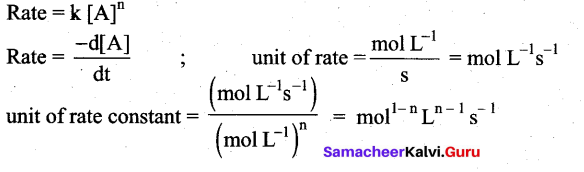In this case, rate = k [Acetone]3/2
n= 3/2
mol1-(3/2)L(3/2)-1s-1 ⇒ mol-(1/2)L(1/2)s-1

Question 8.
Arrange the acids
(i) H2SO3
(ii) H3PO3 and
(iii) HClO3 in the decreasing order of acidity.

(a) (i) > (iii) > (ii)
(b) (i) > (ii) > (iii)
(c) (ii) > (iii) > (i)
(d) (iii) > (i) > (ii)
(d) (iii) > (i) > (ii)
Acidity is directly proportional to oxidation number. As the oxidation number of S, P and Cl in H2SO3, H3PO3 and HClO3 is +4, +3, +5 respectively. So decreasing order of acidity will be (iii) > (i) > (ii)

Question 9.
The value of cell emf of Mercury button cell is –
(a) 1.35 V
(b) – 0.76 V
(c) 0.34 V
(d) 100 V
(a) 1.35 V

Question 10.
In an electrical field, the particles of a colloidal system move towards cathode. The coagulation of the same sol is studied using K2SO4 (i), Na3O4 (ii), K4[Fe(CN)6] (iii) and NaCl (iv). Their coagulating power should be ……….
(a) II > I > IV > III
(b) III > II > I > IV
(c) I > II > III > IV
(d) none of these
(b) III > II > I > IV

Question 11.
Which compound has the highest boiling point?
(a) Acetone
(b) Diethyl ether
(c) Methanol
(d) Ethanol
(d) EthanolQuestion 12.
The reagent used to distinguish between acetaldehyde and benzaldehyde is
(a) Tollens reagent
(b) Fehling’s solution
(c) 2,4 – dinitrophenyl hydrazine
(d) semicarbazide
(b) Fehling’s solution

Question 13.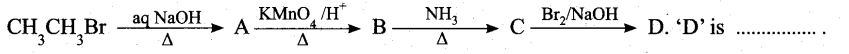(a) bromomethane
(b) a – bromo sodium acetate
(c) methanamine
(d) acetamide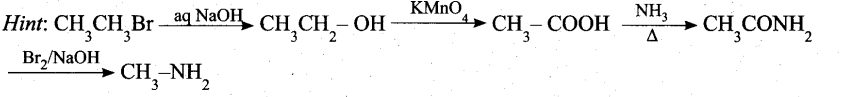(c) methanamine

Question 14.
Match the following.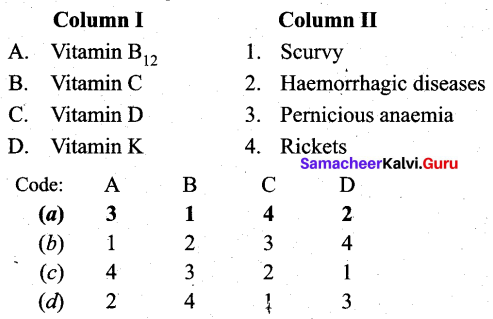(a) A – 3, B – 1, C – 4, D – 2

Question 15.
Regarding cross-linked or network polymers, which of the following statement is incorrect?
(a) Examples are Bakelite and melamine
(b) They are formed from bi and tri-functional monomers
(c) They contain covalent bonds between various linear polymer chains
(d) They contain strong covalent bonds in their polymer chain
(d) They contain strong covalent bonds in their polymer chain

Part – II

Answer any six questions. Question No. 21 is compulsory. [6 × 2 = 12]

Question 16.
Give the uses of zinc.

• Metallic zinc is used in galvanising metals such as iron and steel structures to protect them . from rusting and corrosion.
• Zinc is also used to produce die-castings in the automobile, electrical and hardware industries.
• Zinc oxide is used in the manufacture of many products such as paints, rubber, cosmetics, pharmaceuticals, plastics, inks, batteries, textiles and electrical equipment. Zinc sulphide is used in making luminous paints, fluorescent lights and x-ray screens.
• Brass an alloy of zinc is used in water valves and communication equipment as it is highly resistant to corrosion.

Question 17.
Explain why fluorine always exhibit an oxidation state of-1?
Fluorine the most electronegative element than other halogens and cannot exhibit any positive oxidation state. Fluorine does not have d-orbital while other halogens have d-orbitals. Therefore fluorine always exhibit an oxidation state of-1 and others in halogen family shows +1, +3, +5 and +7 oxidation states.

Question 18.
What is Zeigler -Natta catalyst? In which reaction it is used? Give equation.
A mixture of TiCl4 and trialkyl aluminium is Zeigler – Natta catalyst. It is used in the polymerization.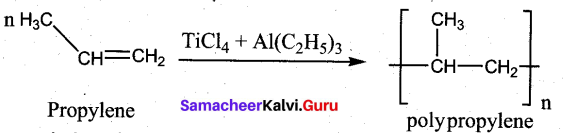Question 19.
Give any three characteristics of ionic crystals.

• Ionic solids have high melting points.
• These solids do not conduct electricity, because the ions are fixed in their lattice positions. ‘
• They do conduct electricity in molten state (or) when dissolved in water because, the ions are free to move in the molten state or solution.

Question 20.
How is surface area of the reactant affect the rate of the reaction?

• In heterogeneous reactions, the surface area of the solid reactants play an important role in deciding the rate.
• For a given mass of a reactant, when the particle size decreases surface area increases. Increase in surface area of reactant leads to more collisions per litre per second and hence the rate of reaction is increased.
• For example, powdered calcium carbonate reacts much faster with dilute HCl than with the same mass of CaCO3 as marble.

Question 21.
Ksp of Al(OH)3 is 1 x 10-15M. At whatpH does 1.0 × 10-3 3M Al3+ precipitate on the addition of buffer of NH4Cl and NH4OH solution?
Al(OH)3 ⇌ Al3+ (aq) + 3OH (aq)
Ksp – [Al3+ ][OH]3
Al(OH)3 precipitates when
[Al3+ ] [OH ]3 > Ksp
(1 × 10-3 )[OH ]3 > 1 × 10-15
[OH]-3] > 1 × 10-12
[OH ] > 1 × 10-4 M
[OH ] = 1 × 10-4 M
pOH = – log1o[OH] = – log(1 × 10-4) = 4
pH = 14 – 4 = 10
Thus, Al(OH)3 precipitates at a pH of 10Question 22.
Arrange the following in the increasing order of their boiling point and give a reason for your ordering: Butan – 2- ol, Butan -l-ol, 2 -methylpropan -2-ol
Boiling points increases regularly as the molecular mass increases due to a corresponding increase in their Van der waal’s force of attraction. Among isomeric alcohols 2°-alcohols have lower boiling points than 1 “-alcohols due to a corresponding decreases in the extent of H-bonding because of steric hindrance. Thus the boiling point of Butan – 2- ol is lower than that of Butan – l-ol. Overall increasing order of boiling points is,
2-methylpropan-2-ol < Butan-2- ol < Butan – l-ol

Question 23.
What are the uses of Benzaldehyde?

• as a flavoring agent
• in perfumes
• in dye intermediates
• as starting material for the synthesis of several other organic compounds like cinnamaldehyde, cinnamic acid, benzoyl chloride etc.

Question 24.
What are anti fertility drugs? Give examples.
Artificially drugs are chemical substances which suppress the action of hormones that promote pregnancy. These drugs actually reduce the chances of pregnancy and act as a protection. Antifertility drugs are made up of derivatives of synthetic progesterone or a combination of derivatives of oestrogen and progesterone.
Example : Ethynylestradiol, menstranol and norethynodrel etc.

Part – III

Answer any six questions. Question No. 30 is compulsory. [6 ×3 = 18]

Question 25.
Give the uses of silicones.
Uses of silicones:

• Silicones are used for low temperature lubrication and in vacuum pumps, high temperature oil baths etc.
• They are used for making water proofing clothes
• They are used as insulting material in electrical motor and other appliances
• They are mixed with paints and enamels to make them resistant towards high temperature, sunlight, dampness and chemicals.

Question 26.
Complete the following reactions.
1. NaCl + MnO2 + H2SO4
2. I2 + S2O32-
3. P4 + NaOH + H2O →
1. 4NaCl + MnO2 + 4H2SO4 → Cl2 + MnCl2 + 4NaHSO4 + 2H2O
2. I2 + 2S2O32- → S4O62- + 2I
3. P4 + 3NaOH + 3H2O → 3NaH2PO2 + PH3

Question 27.
Draw and explain about the structure of chromate and dichromate ion,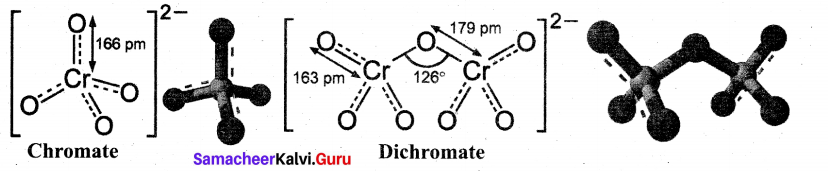• Both chromate and dichromate ions are oxo anions of chromium and they are moderately strong oxidising agents.
• In both structures, chromium is in +6 oxidation state.
• In an aqueous solution, chromate and dichromate ions can be inter convertible, and in an alkaline solution, chromate ion is predominant, whereas dichromate ion becomes predominant in acidic solutions.

Question 28.
Why ionic crystals are hard and brittle?
The ionic compounds are very hard and brittle. In ionic compounds the ions are rigidly held in a lattice because the positive and negative ions are strongly attracted to each other and difficult to separate. But the brittleness of a compound is now easy to shift the position of atoms or ions in a lattice. If we apply a pressure on the ionic compounds the layers shifts slightly. The same charged ions in the lattice comes closer. A repulsive forces arises between same charged ions, due to this repulsions the lattice structure breaks down chemical bonding.Question 29.
Paracetamol is prescribed to take once in 6 hours. Justify this statement.

• Paracetamol is a well known antipyretic and analgesic that is prescribed in cases of fever
and body pain.
• Paracetamol has a half life of 2.5 hours within the body, (i.e) the plasma concentration of the drug is halved after 2.5 hours. So after 10 hours (4 half lives), only 6.25% of drug remains. Based on this, the dosage and frequency will be decided.
• In the case of paracetamol, it is usually prescribed to take once in 6 hours.

Question 30.
A solution of a salt of metal was electrolysed for 150 minutes with a current of 0. 15 amperes. The mass of the metal deposited at the cathode is 0.783g. Calculate the equivalent mass of the metal.
Given, I = 0.15 amperes
t = 150 mins ⇒ t = 150 x 60 sec ⇒ t = 9000 sec
Q = It ⇒ Q = 0.15 x 9000 coulombs ⇒ Q = 1350 coulombs Hence, 135 coulombs of electricity deposit is equal to $$\frac{0.783 \times 96500}{1350}=55.97$$ g of metal.
∴ Hence equivalent mass of the metal is 55.97

Question 31.
What happens when
i. 2 – Nitropropane boiled with HCl
ii. Nitrobenzen electrolytic reduction in strongly acidic medium.
i. 2 – Nitropropane boiled with HCl: 2-nitropropane upon hydrolysis with boiling HCl give a ketone (2-propanone) and nitrous oxide.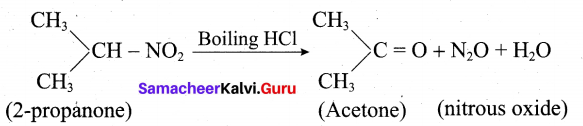ii. Nitrobenezen electrolytic reduction in strongly acidic medium: Electrolytic reduction of nitrobenzene in weakly acidic medium gives aniline but in strongly acidic medium, it gives p-aminophenol obviously through the acid – catalysed rearrangement of the initially formed phenylhydroxylamine.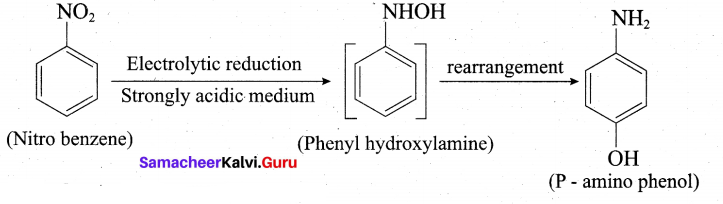Question 32.
Write a short note on peptide bond.

• The amino acids are linked covalently by peptide bonds.
• The carbonyl group of the first amino acid react with the amino group of the second amino acid to give an amide linkage (-CONH) between these aminoacids. This amide linkage is called peptide bond.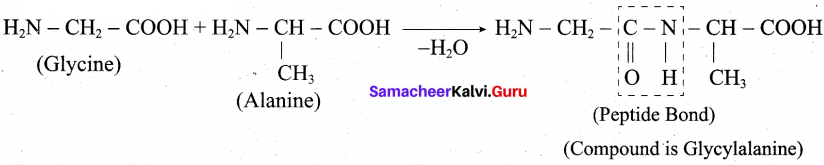• The resulting compound is called a dipeptide. Because, two amino acids are inovlved for getting one peptide bond.
• If large number of amino acids combined through peptide bond, the resulting giant molecule is called a protein.
• The amino end of the peptide is known as N-terminal, while the carboxy end is called C – terminal.Question 33.
(i) What class of drug is Ranitidine?
(ii) If water contains dissolved Ca ions, out of soaps and synthetic detergents, which will you use for cleaning clothes?
(iii) Which of the following is an antisepctic? 0.2% phenol, 1% phenol.
(i) It is an antacid.
(ii) In this case we use synthetic detergents because it give foam with hard water.
(iii) 0.2% solution of phenol acts as antiseptic.

Part – IV

Answer all the questions. [5 × 5 = 25]

Question 34.
(a) (0 Explain the concentration of copper pyrites and galena ores. (3)
(ii) Out of LU(OH)3 and La(OH)3 which is more basic and why? (2)
(b) Explain the preparation of silicones. (5)
(a) (i) Froth floatation: This method is commonly used to concentrate sulphide ores such as galena (PbS), zinc blende (ZnS) etc. In this method, the metallic ore particles which are preferentially wetted by oil can be separated from gangue.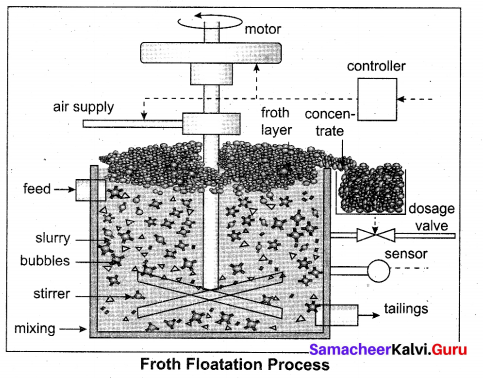In this method, the crushed ore is suspended in water and mixed with frothing agent such as pine oil, eucalyptus oil etc. A small quantity of sodium ethyl xanthate which acts as a collector is also added. A froth is generated by blowing air through this mixture.

The collector molecules attach to the ore particle and make them water repellent. As a result, ore particles, wetted by the oil, rise to the surface along with the froth. The froth is skimmed off and dried to recover the concentrated ore. The gangue particles that are preferentially wetted by water settle at the bottom.

When a sulphide ore of a metal of interest contains other metal sulphides as impurities, depressing agents such as sodium cyanide, sodium carbonate etc are used to selectively prevent other metal sulphides from coming to the froth. For example, when impurities such as ZnS is present in galena (PbS), sodium cyanide (NaCN) is added to depresses the floatation property of ZnS by forming a layer of zinc complex Na2[Zn(CN)4] on the surface of zinc sulphide.

(ii) 1. As we move from Ce3+ to Lu3+, the basic character of Lu3+ ions decreases.
2. Due to the decrease in the size of Lu3+ ions, the ionic character of Lu – OH bond decreases, covalent character increases which results in the decrease in the basicity.
3. Hence, La(OH)3 is more basic than Lu(OH)3.

[OR]

(b) Generally silicones are prepared by the hydrolysis of dialkyldichlorosilanes (R2SiCl2 ) or diaryldichlorosilanes Ar2SiCl2, which are prepared by passing vapours of RCl or ArCl over silicon at 570 K with copper as a catalyst.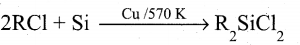The hydrolysis of dialkylchloro silanes R2SiCl2 yields to a straight chain polymer which grown from both the sides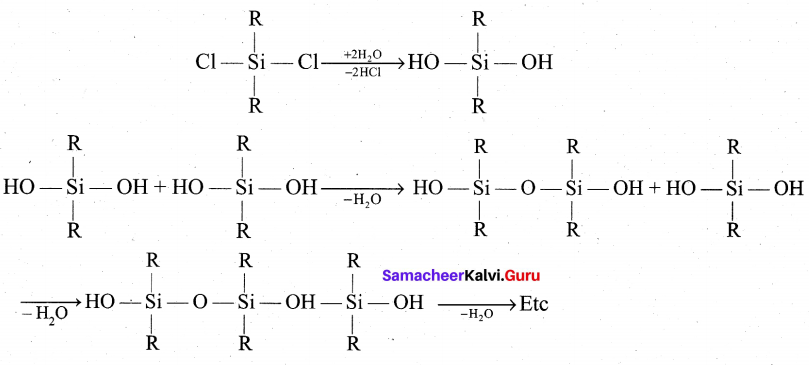The hydrolysis of monoalkylchloro silanes RSiCl3 yields to a very complex cross linked polymer. Linear silicones can be converted into cyclic or ring silicones when water molecules is removed from the terminal -OH groups.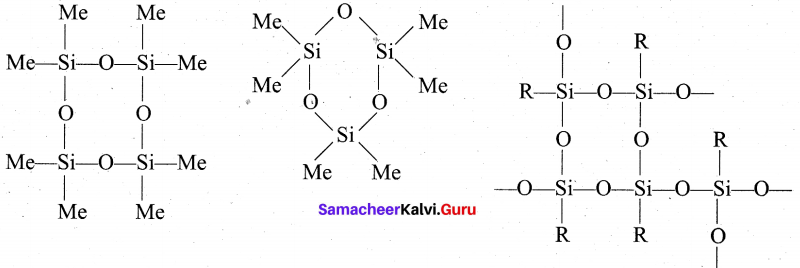Question 35.
(a) Bleaching action of chlorine is permanent – Justify this statement and also give the uses of chlorine. (5)
[OR]
(b) (i) Complete the following (3)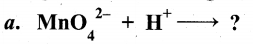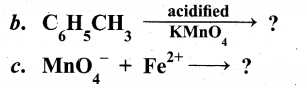(ii) What is linkage isomerism? Explain with an example.
(a) Chlorine is a strong oxidising and bleaching agent because of the nascent oxygen.
H2O + Cl2 → HCl + HOCl (Hypo chlorous acid)
HOCl → HCl + (O)

Colouring matter + Nascent oxygen → Colourless oxidation product
Therefore, bleaching of chlorine is permanent. It oxidises ferrous salts to ferric, sulphites to sulphates and hydrogen sulphide to sulphur.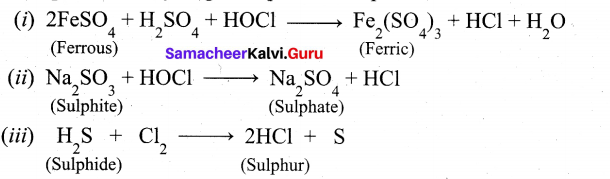Uses of chlorine:

• Purification of drinking water
• Bleaching of cotton textiles, paper and rayon
• It is used in extraction of gold and platinum

[OR]

(b) (i)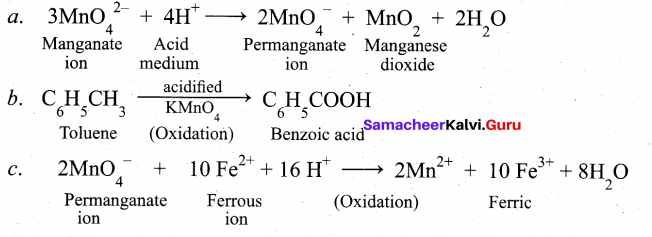(ii) This type of isomers arises when an ambidentate ligand is bonded to the central metal atom/ion through either of its two different donor atoms.
For examples,
[CO(NH3)5ONO]Cl2
(Pentaammine nitrito cobalt (III) chloride)
O – attached. (Red in colour).
[CO(NH3)5NO2]Cl2
(Pentaammine nitro cobalt (III) chloride)
N – attached (Yellow-brown in colour).Question 36.
(a) (i) What is the two dimensional coordination number of a molecule in square close packed layer? (2)
(ii) Derive the integrated rate law for a first order reaction? (3)
[OR]
(b) (i) Define solubility product. (2)
(ii) What is the pH of an aqueous solution obtained by mixing 6 gram of acetic acid and 8.2 gram of sodium acetate and making the volume equal to 500 ml. (Given: Ka for acetic acid is 1.8 × 10-5) (3)
(a) (i) Square close packing : When the spheres of the second ” row are placed exactly above those of the first row.
This way the spheres are aligned horizontally as well as vertically. The arrangement is AAA type. Coordination number is 4.
(ii) Areaction whose rate depends on the reactant concentration raised to the first power is called a first order reaction.
First order reaction is A → product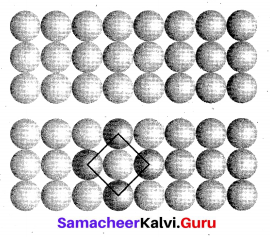Rate law can be expressed as, Rate = k [A]1
Where, k is the first order rate constant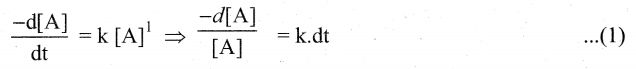Integrate the above equation (1) between the limits of time t = O and time equal to t,
while the concentration varies from initial concentration [A0]to [A] at the later time.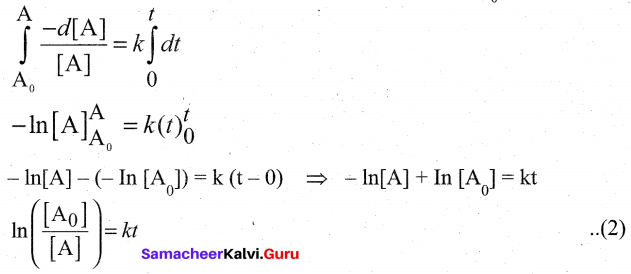This equation (2) is in natural logarithm. To convert it into usual logarithm with base 10,
we have to multiply the term by 2.303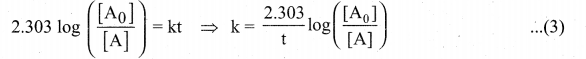[OR]

(b) (i) Solubility product : It is defined as the product of the molar concentration of the constituent ions, each raised to the power of its stoichiometric co-efficient in a balanced equilibrium equation.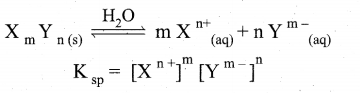(ii) According to Henderson — Hessalbalch equation,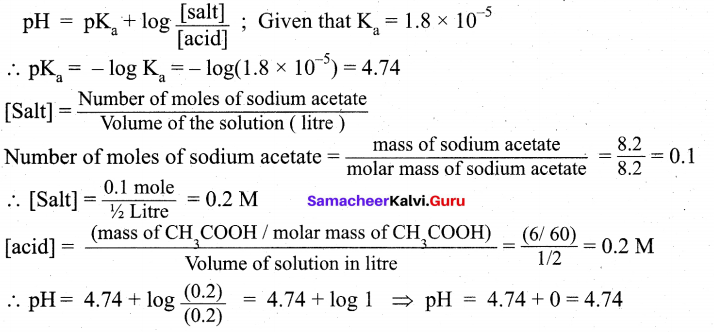Question 37.
(a) (i) Why for CH3COOH cannot be determined experimentally? (2)
(ii) Write about the classification of organic nitro compounds. (3)
[OR]
(b) Describe about condensation methods of preparation of colloids. (OR)
Describe chemical methods of preparation of colloids. (5)
(a) (i) Molar conductivity of weak electrolytes keeps on increasing with dilution and does not
become constant even at very large dilution.

(ii)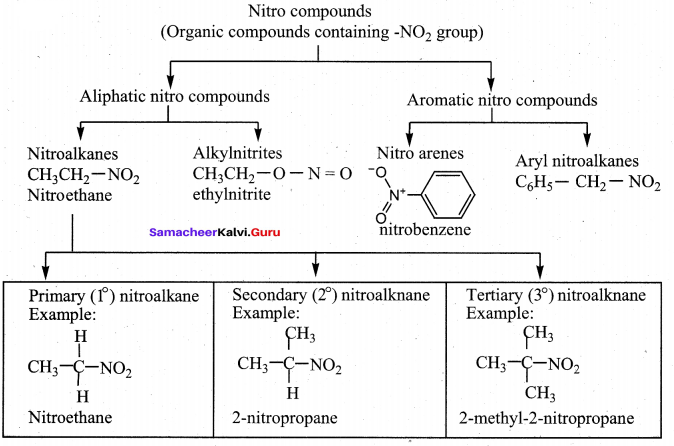[OR]

(b) When the substance for colloidal particle is present as small sized particle, molecule or ion, they are brought to the colloidal dimension by condensation methods.
i. Oxidation method:- When hydroiodic acid is treated with iodic acid I sol is obtained.
HIO3 +5HI → 3H2O + 3I 2(sol)

ii. Reduction method:- Gold sol is prepared by reduction of auric chloride using formaldehyde.
2 AuCl3 + 3 HCHO + 3 H2O (sol) → 2 Au(sol) + 6HCl + 3HCOOH

iii. Hydrolysis:- Ferric chloride is hydrolysed to get ferric hydroxide colloid
FeCl3 + 3H2O → Fe(OH)3 (sol) + 3HCl

iv. Double decomposition:- When hydrogen sulphide gas is passed through a solution of arsenic oxide, a yellow coloured arsenic sulphide is obtained as a colloidal solution.
As2O3 + 3H2 → As 2S3 + 3H2O

v. Decomposition:- When few drops of an acid is added to a dilute solution of sodium thiosulphate, sulphur colloid is produced by the decomposition of sodium thio sulphate.
S2O32- + 2H+ → S(sol) + H2O + SO2

Question 38.
(a) (i) What is Clemmensen reduction ? Explain it. (2)
(ii) Write the structure of the major product of the aldol condensation of benzaldehyde
with acetone. (3)
[OR]
(b) (i)How will you convert nitrobenzene into (2)
1. 1,3, 5 – trinitrobenzene 2. o añd p- nitrophenol
(ii) Differentiate between Globular and fibrous proteins. (3)
(a) (i) Aldehyde and ketones when heated with zinc amalgam and concentrated hydrochloric acid give hydrocarbons. This reaction is known as Clemmensen reduction.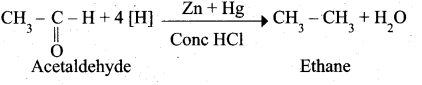(ii) Aldol condensation of benzaldehyde with acetone: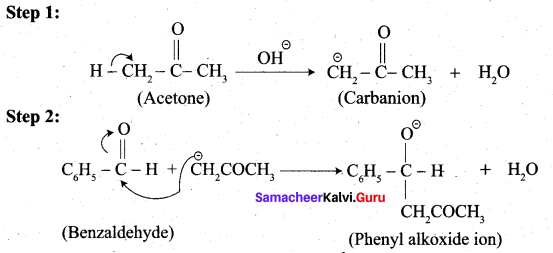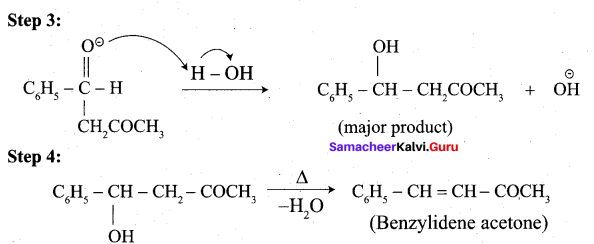(b) (i) 1. Conversion of nitrobenzene into 1,3,5 – trinitrobenzene: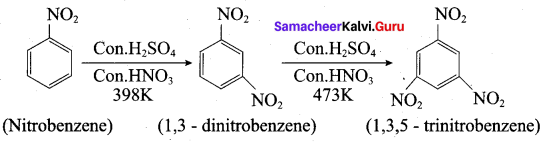2. Conversion of nitrobenzene into o and p- nitrophenol:
Nitrobenzene heated with solid KOH at 340 K gives a low yield of a mixture of O-and P-nitrophenols.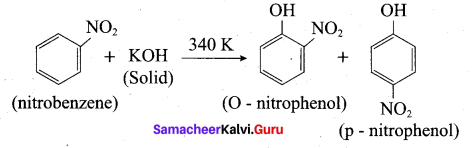(ii) Difference between Globular and fibrous proteins.

 No. Globular proteins Fibrous Proteins i They form a-helix structure They have (3-pleated structure. ii They are soluble in water: They are insoluble in water. iii They are cross lined condensation polymers of acidic and basic amino acids. They are linear condensation polymeric proteins.